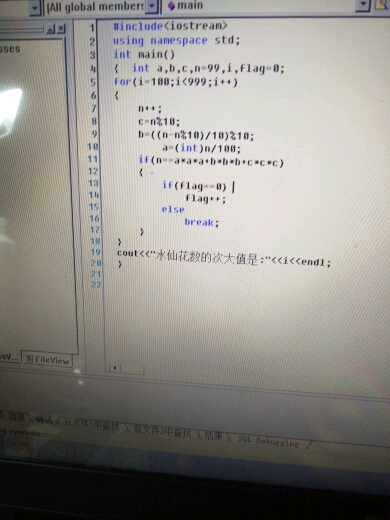###### ji1812062521

2015-12-11 11:15 阅读 2.2k

# 3位正整数的全部水仙花数的次大值• 点赞
• 写回答
• 关注问题
• 收藏
• 复制链接分享

#### 3条回答默认 最新

• 已采纳
``````include"iostream"
using namespace std;

void main()
{
int a,b,c,i,n1,n2;

n1=n2=0;
for( i=100; i<=999; i++ )
{
c=i%10;
b=(i-i%10)/10%10;
a=i/100;
if( i == a*a*a+b*b*b+c*c*c )
{
n1=n2;//n1是次大值，n2是最大值
n2=i;
}
}
cout<<"水仙花的次大值是："<<n1<<endl;
}
``````
点赞 评论 复制链接分享
• 怎么错了点赞 评论 复制链接分享
• 筛选次大不应该从大往小搜吗？
‘/’可以自动转换成int型，不需要强制转换
还有最后一件，i只存在在循环里，循环外没有i这个变量

``````
#include<iostream>
using namespace std;
int main(){
int a=1,b=0,c=0;
bool flag=0;
while(!flag&&a*a*a+b*b*b+c*c*c!=a+100+b*10+c){
c++;
if(c>=10){b++;c=0;}
if(b>=10){a++;b=0;}
}
cout<<"水仙花的次大值是："<<a<<b<<c;
}
``````
点赞 评论 复制链接分享